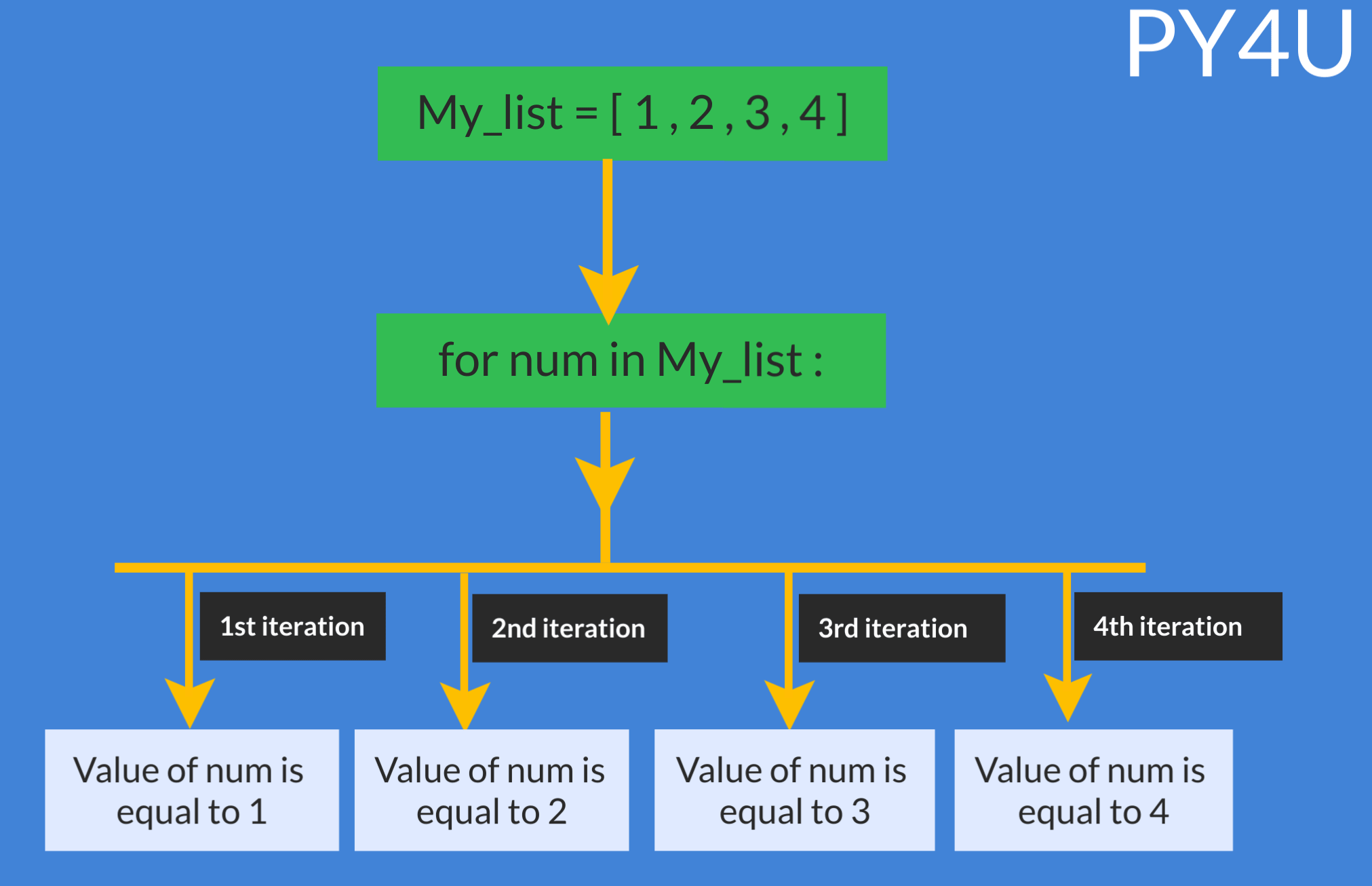Loops In Python

Python has two types of loops :

• for loop
• while loop

The two loops are very specific in their use. for loops are often used for iterations over an object or a data structure . Like every character in a string or every object in a list. while loops are often used when the initial condition is known and a specific block of code is to be executed until that condition becomes false.

for loop:

Let us understand Iteration Through For loop.Note that you have full flexibility over choosing the name of the variable in for loop. Instead of my_var , you can choose cat , jelly , or even just 'x', anything you like. However it is a good practice to choose a name that is somehow related to objects or elements. Like , for instance , if your dealing with integers , the most basic variable name would have been 'num'.

Let us take some examples based on for loops.

my_list = [1,2,3,4,5,6]
for x in my_list:
print(x)

for x in my_list :
print("hello world")
output :
1
2
3
4
5
6
hello world
hello world
hello world
hello world
hello world
hello world

Let us use for loop along with conditional statements . In the example below i have some numbers in my_list and i want to print only the even numbers.

my_list = [1,2,3,4,5,6]
for num in my_list :
# modulo operator would give the remainder of the division
if num % 2 ==0:
print(num)
output :
2
4
6

Let us make use of a counter to calculate the sum of all the elements in a list. The counter should be assigned a value of zero initially and every element should be added to it every time a iteration is done. Let us see an example to understand this.

my_list = [1,2,3,4,5,6]
my_sum = 0
for x in my_list :
my_sum = my_sum + x
print(my_sum)
output :
21

Let us go ahead and iterate through a string and a tuple.

for x in "PY4U.net":
print(x)
my_tuple = ( 1,2,3 )
for x in my_tuple :
print(x)
output :
P
Y
4
U
.
n
e
t
1
2
3
while loop :

while loop executes a block of code while a condition is true . Syntax of the while loop is as follows:

• while Some_boolean_condition :
# block of code to be executed.

Let us take an example based on while loop. In the example below , we have to print all the numbers less than 7 using while loop. In such problems it is essential to use a counter such that when the value of counter becomes more than or equal to 7 , the loop should get terminated. Let us see the code below.

#always assign zero to your counter
x = 0
while x < 7 :
print(x)
x = x+1

output :
0
1
2
3
4
5
6
Nested Loops

Sometimes it is required to use single or multiple loop(s) within a single parent loop. In the example given below , we have two lists and we want to store the product of each elements into a third list.

list_1 = [1,2,3,4]
list_2 = [5,6,7]
list_3 = []

for x in list_1:
for y in list_2:
list_3.append(x*y)

print(list_3)
[5, 6, 7, 10, 12, 14, 15, 18, 21, 20, 24, 28]

Popular Tutorials